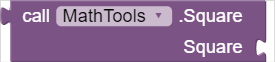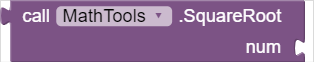# MathTools

This is a simple math tools. Developed by Sabpab Creator:

💡  Current Version 1.0
📁  File Size 5.91 KB
📦  com.sabpabcreator.mathtools.MathTools
📅   Created On 2021-08-28

## Method Blocksa input type number
b input type numberDivide - Divide
a input type number
b input type numberMultiply - Multiply
a input type number
b input type numberPercentage - Percentage
percent input type number
of input type numberSquare - Square
Square input type numberSquareRoot - Square Root
num input type numberSubstraction - Substraction
a input type number
b input type number

This docs is generated from AIX Docs - Cttricks. Hitbutton if you liked this extension and feel free to comment below your reviews and suggestion.

6 Likes

Nice extension keep it up

1 Like

Thank You Very Much, This is my first extension.

2 Likes

Congrats for the first extension bro1 Like

I generated these blocks from here - https://cttricks.com/aix-docs/ and Thank You